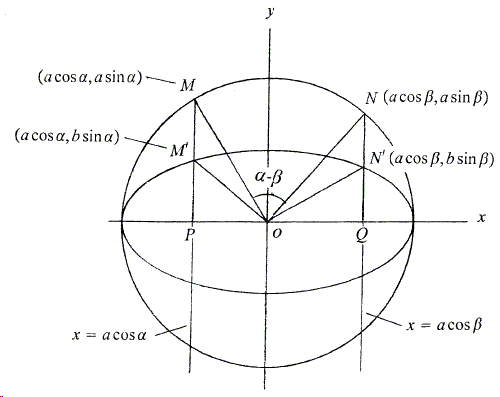A Generalized Cavalieri-Zu Principle
Sidney Kung

# Area, Sector Area, and Segment Area of an EllipseLet $A_{1}$ and $A_{2}$ be the areas of a circle and an ellipse, respectively. Line $x=\mbox{cos}\theta$ intersects the circle at $A$, $B$ and the ellipse at $A',$ and $B'$, respectively. It's easy to see that $\frac{A'C}{AC}=\frac{A'B'}{AB}=\frac{b}{a}$. Thus, from (*), the area of the ellipse is

(1)

$A_{2}=\frac{b}{a}A_{1}=\frac{b}{a}\pi a^{2}=\pi ab$.

An elliptic sector is a region bounded by an arc and line segments connecting the center of the ellipse (the origin in our diagrams) and the endpoints of the arc.Let lines $x=a\space\mbox{cos}\alpha$ and $x=a\space\mbox{cos}\beta$ be perpendicular to the $x$-axis, and let $[F]$ indicate the area of figure $F$.

The coordinates of the points $M$, $M'$, $N$, $N'$ are $(a\space\mbox{cos}\alpha , a\space\mbox{sin}\alpha)$, $(a\space\mbox{cos}\alpha , b\space\mbox{sin}\alpha)$, $(a\space\mbox{cos}\beta , a\space\mbox{sin}\beta)$, and $(a\space\mbox{cos}\beta , b\space\mbox{sin}\beta)$, respectively. Since $\frac{N'Q}{NQ}=\frac{M'P}{MP}=\frac{b}{a}$, we have $\frac{[PM'N'Q]}{[PMNQ]}=\frac{[N'OQ]}{[NOQ]}=\frac{[M'OP\space]}{[MOP\space]}=\frac{b}{a}$. Thus,

$\frac{[PM'N'Q]-[N'OQ]-[M'OP\space]}{[PMNQ]-[NOQ]-[MOP\space]}=\frac{b}{a}$, or $\frac{[M'ON']}{[MON\space]}=\frac{b}{a}$.

Therefore, the area of the elliptic sector $M'ON'$ is

(2)

$[M'ON']=\frac{b}{a}\left(\frac{\alpha -\beta}{2\pi}\right)\pi a^{2}=\frac{1}{2}(\alpha -\beta)ab$. $(\alpha \gt \beta)$.

An elliptic segment is a region bounded by an arc and the chord connecting the arc's endpoints.Note that the area of the elliptic segment (in then diagram) is equal to the area of sector $M'ON'$ minus the area of $\triangle M'ON'$. Hence, the elliptic segment area is

(3)

$\frac{ab}{2}(\alpha -\beta)-\frac{b}{a}\left(\frac{a^{2}}{2}\mbox{sin}(\alpha -\beta)\right) =\frac{ab}{2}\left((\alpha-\beta)-\mbox{sin}(\alpha-\beta)\right)$.